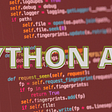# An Easy Example to Show How the Recurse Work

• for digit set [1,2,…,9], find k numbers that sum up to n. Each number is used at most once:
`Input: k = 3, n = 7Output: [[1,2,4]]Explanation:1 + 2 + 4 = 7`
`      ...[1,2][1,3][1,4]...[1,9][1,2,3][1,2,4],[1,2,5]...[1,2,9]...[2,3][2,4][2,5]...[2,9][2,3,4][2,3,5][2,3,6]...[2,3,9]...[3,4][3,5][3,6]...[3,9][3,4,5][3,4,6][3,4,7]...[3,4,9]...`
`def recurse_sum(S,digit):  for d in range(digit,10):    recurse_sum(S+[d],d+1)recurse_sum([],1)`
`def combinationSum3(self, k: int, n: int) -> List[List[int]]:        ans = set()        def recurse_sum(S:List[int], digit:int ) -> None:            #print(f"S={S}, digit={digit}")            if len(S) == k and sum(S)== n:                ans.add(tuple(S))                return            if len(S) > k:                return            for d in range(digit, 10):                recurse_sum(S+[d], d+1)            return        recurse_sum([],1)        return ans`

## Let's have another example:

` candidates = [2,3,6,7], target = 7`
`[[2,2,3],]`
`combination      target      remain#1                 7          5#2[2,2]               7          3#3[2,2,2]             7          1#4[2,2,2,2]           7         -1  return to next#5[2,2,2,3]           7         -2  return to next#6[2,2,2,6]           7         -5  return to next#7[2,2,2,7]           7         -6  return to #3.next=#8#8[2,2,3]             7          0  return to next#9[2,2,6]             7         -3  return#10[2,2,7]            7         -4  return to #2.next=#11#11[2,3]              7          2#12[2,3,3]            7         -1  return to #11.next=#13#13[2,6]              7         -1  return to #13.nezt=#14#14[2,7]              7         -2  return to #1.nezt=#15#15                7         4#16[3,3]              7         1#17[3,3,3]            7         -2#18[3,3,6]            7         -8`
`def recurse_sum(S, remain, start_i):  if remain == 0:     res.add(S)     return  if remain < 0:      return  for i in range(start_i,len(candidates)):     recurse_sum(S+[candidates[i],remain-candidate[i], i+1)`
`     #recurse_sum([]+[candidates[i],remain-candidate[i], i+1)     tmpS = S+[candidates[i]]     recurse_sum(tmpS, target - sum(tmpS), i)`
`def combinationSum(self, candidates: List[int], target: int) -> List[List[int]]:        res = set()        def recurse_sum(S:List[int], remain:int, start_i:int)->None:            if remain == 0:                res.add(tuple(S))                return            if remain < 0:                return            for i in range(start_i,len(candidates)):               # recurse_sum(S+[candidates[i]], remain - candidates[i], i)                tmpS = S+[candidates[i]]                recurse_sum(tmpS, target - sum(tmpS), i)        recurse_sum([],target,0)         return res`

--

--

--

## More from Paul Xiong

Coding, implementing, optimizing ML annotation with self-supervised learning, TLDR: doctor’s labeling is the 1st priority for our Cervical AI project.

Love podcasts or audiobooks? Learn on the go with our new app.

## Study Guide: AZ-500 — Microsoft Azure Security Technologies## Summer Internship-Online Examination Based Web Application## Get Palm Oil Rates Using Python API## Rocketswap Missions Competition## Switch Statements to the Rescue## Feathering for SSIDs.## Paul Xiong

Coding, implementing, optimizing ML annotation with self-supervised learning, TLDR: doctor’s labeling is the 1st priority for our Cervical AI project.

## How To Install TensorFlow Version 2.8 on The M1 chip Macbook With Ease## How to process and plot Audio data with Python## Generate art using aggdraw and Python — Part 1